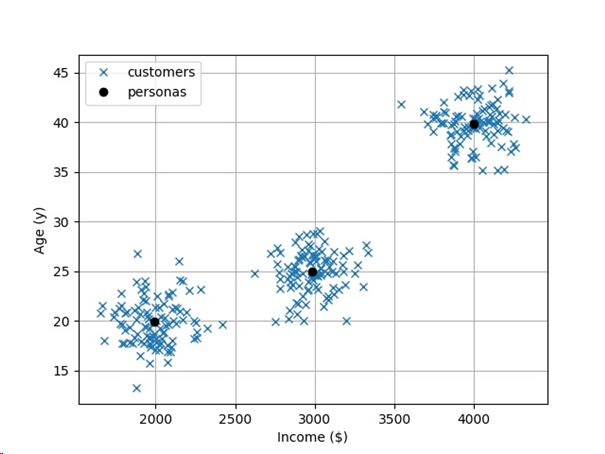[Tutorial] How to Run K-Means Clustering in 1 Line of Python?

If there is one clustering algorithm you need to know – whether you are a computer scientist, data scientist, or machine learning expert – it’s the k-Means algorithm. In this section, you’ll learn the general idea and when and how to use it in a single line of Python code.

You may know about supervised learning where the training data is “labeled”, i.e., we know the output value of every input value in the training data. But in practice, this is not always the case. What if you have “unlabeled” data? Especially in many data analytics applications, there is no such thing as “the optimal output”. Prediction is not the goal here – but you can still distill useful knowledge from these unlabeled data sets.

The Basics

For example, suppose you are working in a startup which serves different target markets with various income levels and ages. Your boss tells you to find a certain number of target “personas” that best fit your different target markets.

It’s time to learn about “unsupervised learning” with unlabeled training data. In particular, you can use clustering methods to identify the “average customer personas” which your company serves.

Here is an example:

Visually, you can easily see three types of Personas with different types of incomes and ages. But how to find those algorithmically? This is the domain of clustering algorithms such as the widely popular K-Means algorithm.

Given the data sets and an integer k, the K-Means algorithm finds k clusters of data such that the difference between the k cluster centers (=the centroid of the data in each cluster) and the data in the k cluster is minimal.

In other words, we can find the different personas by running the K-Means algorithm on our data sets:

The cluster centers (black dots) fit very nicely to the overall data. Every cluster center can be viewed as one customer persona. Thus, we have three idealized personas:

• A 20-year-old earning \$2000,
• A 25-year-old earning \$3000, and
• A 40-year-old earning \$4000.

And the great thing is that the K-Means algorithm finds those cluster centers completely automated – even in a high-dimensional space (where it would be hard for humans to find the personas visually).

As a small side note: The K-Means algorithm requires “the number of cluster centers k” as an input. In this case, we use domain knowledge and “magically” defined k=3. There are more advanced algorithms that find the number of cluster centers automatically.

So how does the K-Means algorithm work? In a nutshell, it performs the following procedure:

1. Initialize random cluster centers (centroids).
2. Repeat until convergence
• Assign every data point to its closest cluster center.
• Recompute each cluster center to the centroid of all data points assigned to it.

The Code

How can we do all of this in a single line of code? Fortunately, the Scikit-learn library in Python has already implemented the K-Means algorithm in a very efficient manner. So here is the one-liner that does K-Means clustering for you:

## Dependencies
from sklearn.cluster import KMeans
import numpy as np

## Data (Work (h) / Salary (\$))
X = np.array([[35, 7000], [45, 6900], [70, 7100],
[20, 2000], [25, 2200], [15, 1800]])

## One-liner
kmeans = KMeans(n_clusters=2).fit(X)

## Result & puzzle
cc = kmeans.cluster_centers_
print(cc)

Python Puzzle: What’s the output of this code snippet? Try to guess a solution without understanding every syntactical element!

(In the next paragraphs, I will give you the result of this code puzzle. In my opinion, puzzle-based learning is one of the best way to acquire the basics in programming. That’s why I have written the book “Coffee Break Python” to learn Python faster — and to fit learning in any daily schedule.)

The Results

In the first lines, we import the KMeans module from the sklearn.cluster package. This module takes over the clustering itself. Also, we need to import the NumPy library because the KMeans module works on NumPy arrays.

The data is two-dimensional. It correlates the number of working hours with the salary of some workers. There are six data points in this employee data set:

The goal is to find the two cluster centers that fits best to this data.

## One-liner
kmeans = KMeans(n_clusters=2).fit(X)

In the one-liner, we explicitly define the number of cluster centers using the function argument n_clusters. First, we create a new KMeans object that handles the algorithm for us. We simply call the instance method fit(X) to run the K-Means algorithm on our input data X. The KMeans object now holds all the results. All which is left is to retrieve the results from its attributes.

cc = kmeans.cluster_centers_
print(cc)

So, what are the cluster centers and what is the output of this code snippet?

In the graphic, you can see that the two cluster centers are (20, 2000) and (50, 7000). This is also the result of the Python one-liner.

Where to go from here?

In this article, you have learned how to run the popular K-Means algorithm in Python — using only a single line of code.

I know that it can be hard to understand Python code snippets. Every coder is constantly challenged by the difficulty of code. Don’t let anybody tell you otherwise.

To make learning Python less of a pain, I have created a Python cheat sheet course where I’ll send you a concise, fresh cheat sheet every week. Join my Python course for free!